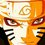# Olympiad proof Problem - Day 1

From this day onwards I am going to post some proof problems.So that you can use these as practice problems for Olympiads. Let us start from basic level (not very basic though).

Find the minimum value of the expression below

$\dfrac{a}{b+c+d} + \dfrac{b}{a+c+d} + \dfrac{c}{a+b+d} + \dfrac{d}{a+b+c}$

Details and Assumptions:

• $a$, $b$, $c$ and $d$ are positive real numbers.

Try to make different approaches.

Try more proof problems at Olympiad Proof Problems.Note by Surya Prakash
5 years, 8 months ago

This discussion board is a place to discuss our Daily Challenges and the math and science related to those challenges. Explanations are more than just a solution — they should explain the steps and thinking strategies that you used to obtain the solution. Comments should further the discussion of math and science.

When posting on Brilliant:

• Use the emojis to react to an explanation, whether you're congratulating a job well done , or just really confused .
• Ask specific questions about the challenge or the steps in somebody's explanation. Well-posed questions can add a lot to the discussion, but posting "I don't understand!" doesn't help anyone.
• Try to contribute something new to the discussion, whether it is an extension, generalization or other idea related to the challenge.

MarkdownAppears as
*italics* or _italics_ italics
**bold** or __bold__ bold
- bulleted- list
• bulleted
• list
1. numbered2. list
1. numbered
2. list
Note: you must add a full line of space before and after lists for them to show up correctly
paragraph 1paragraph 2

paragraph 1

paragraph 2

[example link](https://brilliant.org)example link
> This is a quote
This is a quote
    # I indented these lines
# 4 spaces, and now they show
# up as a code block.

print "hello world"
# I indented these lines
# 4 spaces, and now they show
# up as a code block.

print "hello world"
MathAppears as
Remember to wrap math in $$ ... $$ or $ ... $ to ensure proper formatting.
2 \times 3 $2 \times 3$
2^{34} $2^{34}$
a_{i-1} $a_{i-1}$
\frac{2}{3} $\frac{2}{3}$
\sqrt{2} $\sqrt{2}$
\sum_{i=1}^3 $\sum_{i=1}^3$
\sin \theta $\sin \theta$
\boxed{123} $\boxed{123}$

Sort by:

By using $A.M-H.M$ we get , $\dfrac{\displaystyle\sum_{cyc}^{a,b,c,d}\left(\frac{a}{b+c+d}+1\right)}{4}\geq\frac{4}{\sum_{cyc}^{a,b,c,d}\left(\dfrac{1}{1+\dfrac{a}{b+c+d}}\right)}$ $\displaystyle\sum_{cyc}^{a,b,c,d}\left(\dfrac{a}{b+c+d}+1\right)\geq\dfrac{16}{3}$ $\displaystyle\sum_{cyc}^{a,b,c,d}\left(\dfrac{a}{b+c+d}\right)\geq\dfrac{16}{3}-4$ $\displaystyle\sum_{cyc}^{a,b,c,d}\left(\dfrac{a}{b+c+d}\right)\geq\dfrac{4}{3}$

- 5 years, 8 months ago

Nice solution. Do you have any more approaches?

- 5 years, 8 months ago

Generalization:

$\Large{\sum_{i=1}^n}\large{ \dfrac{a_i}{\small{\displaystyle\sum_{j=1}^{n}a_{j}-a_i}}\geq \dfrac{n}{n-1}}$

When $n=3$ we get Nesbitt's inequality and when $n=4$ we get the inequality of this note.

- 5 years, 8 months ago

Proof:

$\sum_{cyc} \dfrac{a}{\sum_{cyc} a - a}+n -n$ $=\sum_{cyc} (\dfrac{a}{\sum_{cyc} a - a}+1) -n$ $=\sum_{cyc} (\dfrac{\sum_{cyc} a}{\sum_{cyc} a - a} -n$ $=\sum_{cyc} a [\dfrac{1}{\sum_{cyc} a - a}] -n$ Titu's Lemma/Cauchy-Shwarz in engel form$\geq \sum_{cyc} a [\dfrac{n^2}{(n-1)\sum_{cyc} a}]-n$ $=\dfrac{n^2}{n-1} -n$ $=\dfrac{n}{n-1}$

- 5 years, 8 months ago

@Nihar Mahajan nice idea..... how did you reach at this generalization?

- 5 years, 8 months ago

When I was proving this inequality , I felt a bit restricted , hence gave the generalization

- 5 years, 8 months ago

So, here is my solution.

The given expression is homogeneous equation of degree $0$. So, we can make a constraint $a+b+c+d=1$. And this constraint gives us that $0 < a,b,c,d <1$.

The expression transforms into $\sum_{a,b,c,d} \dfrac{a}{1-a}$

Let $f(x) = \dfrac{x}{1-x}$. Then we get that $f''(x) = -2(x-1)^{-3} > 0$ for all $x<1$.

It implies that

$f(a) + f(b) + f(c) + f(d) \geq 4f\left(\dfrac{a+b+c+d}{4}\right) = 4 f \left(\dfrac{1}{4}\right) = \dfrac{4}{3}$.

- 5 years, 8 months ago

We can also solve it using Titu's Lemma. I am currently out of station. I would post the proof as soon as I return home.

- 5 years, 8 months ago

1) put all 4 equal. To get minimum as 4/3. 2)put deniminator of all fractions as w,x,yand z. Now find each a,b,c and in terms of w,x,y and z. All these (w,x,y and z )are positive real no.s. now apply AM>=GM . 3) Rather finding out each a to d in terms of w,x,y and z , one can add +1 to each term take (a+b+c+d) common apply AM>=GM . Later substract 4 from answer

- 5 years, 8 months ago

'put all 4 equal. To get minimum as 4/3'.. WHY?

- 5 years, 8 months ago

How can you say that minimum occurs when all the four quantities are equal? You should prove that.

- 5 years, 8 months ago

What if we get maximum when all 4 are equal?

- 5 years, 8 months ago